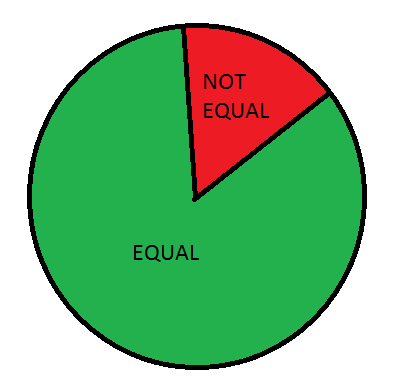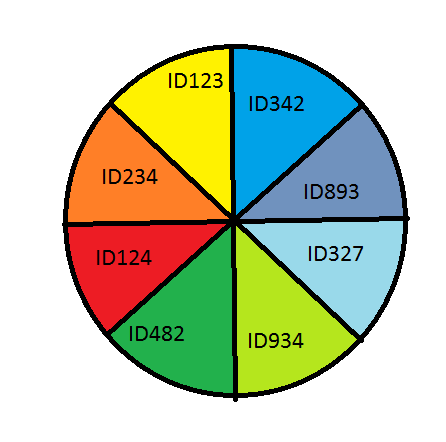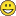# App Development

Announcements
Qlik Insider: SaaS capabilities for Data Integration and Analytics, May 25th! Register Now
cancel
Showing results for
Did you mean:Contributor III

## Pie Chart with 2 Measures?

Hello everyone,

first of all: I am so happy to have this community, because otherwise I would probably start hating my work. So, a huge thank you to everyone here who keeps answering questions and trying to help people make sense of this program. I'm having a terrible day, so I'm sorry for any unhappy sentiments towards Qlik.

Now to my sad little problem:

I'm working on translating a Qlik View App to Qlik Sense right now. One of the charts is a pie chart containing two measures, which, sadly but obviously, doesn't work in Qlik Sense.

This is what the measures do:

= num(

count(

distinct

IF(Interest_Rate = 0

AND End_Date-1 = To_Date AND Row_Nr=1, LoanID&PartID)

)

)

The second one just checks whether the Interest Rate is NOT 0. So it's just looking for equals vs. is different.

I tried pasting that into a QSense pie chart dimension, but there I'm getting a random "Invalid dimension" error (isn't it great how Qlik Sense, in contrast to programs like R or Python, doesn't tell you what the problem is or where it is, just that there is one?).

I've already looked around here and seen that people use the Aggr function to avoid..something..(?) that makes it report the 'Invalid dimension' error, but I can't get that to work either - I've seen people put in a new field at the end of the expressions, and I don't know what the specific field is supposed to be. (Like here: Aggr(If(Count(DISTINCT EMAIL) > 3, '4 and more', '3 and less'), Client) from https://community.qlik.com/thread/226408)

I would be so grateful for some thoughts & tips as to how I can get this stupid pie chart to work!

Thank you everyoneCinta

1 Solution

Accepted SolutionsMVP

For the sample provided, is there no place where Interest Rate = Interest Component? If not, then try this

Dimension

=Aggr(If(Interest_Rate = 0 AND End_Date-1 = To_Date AND Row_Nr=1, 'Interest Rate = Interest Components', 'Interest Rate <> Interest Components'), ID)

Expression

=Count(DISTINCT ID)

18 RepliesMVP

Why are you adding the above as a dimension? Why not as a measure? I think the error is because you tried to add it as a dimension... once you add it as a measure, you should be fineContributor III
Author

Hey Sunny,

well, I would need it as 2 measures.. and that doesn't work for a pie chart. If I try to add it as one measure defining an if statement with = 0 or <> 0, I need something to fill it - and there the dimension then doesn't fill by equal or unequal, but something like ID, and that doesn't represent what I'm trying to do.MVP

I don't fully understand, but may be omarbensalem‌ have any ideasContributor III
Author

I'm sorry! I'll try it again:

I'm looking for something like this:whereas, when I add the above as a measure & fill the dimension with ID, I get something like this:which is obviously not very helpful.Meaning, the dimension lets me define what I want the pie chart to show - unequal / equal or just an arbitrary ID. I need it to show what amount of the data is equal vs unequal, the IDs don't interest me.Partner

as dimension:

ValueList('Equal','Not Equal')

as measure:

if( ValueList('Equal','Not Equal')='Equal', the measure u're using for equal,

if( ValueList('Equal','Not Equal')='Not Equal', the measure u're using for not equal ))

Hoê this helps !Contributor III
Author

Hey! Thank you for your replyWhat does ValueList mean here? Do I replace it with the rest of the expression? Or do I replace some other part? I'm confused, but I want to use it!MVP

So, think about it this way.... which field are you going to use in order to see the result of the below expression and then use the numbers in your if statement?

Count(DISTINCT If(Interest_Rate = 0 and End_Date-1 = To_Date and Row_Nr = 1, LoanID&PartID)

For example, I Count(DISTINCT Employee) against Region field

Region     Count(DISTINCT Employee)

NA               1400

EUROPE     2000

....

....

Now I want to categorize this count by > 1500 and <=1500. So, I know that the field that I need is Region. I can add more dimensions, such as add Country to get a more detailed count. What is your dimension here?Partner

Hi Cinta,